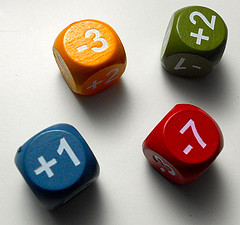# Mathematics: Getting started

Mathematics support for students at the University of Suffolk.

## Numeracy## Getting your maths up to speed and improving your numeracy skills

• Multiplication and Division
• Decimals
• Fractions
• Ratios
• Percentages
• Measurements
• Mathematical Language

Mathematical symbols and language (PDF from Mathcentre)

We can also offer you a great course through our lynda.com subscription, available to all students, on Everyday Math Fundamentals

## Resources for Staff

Members of staff who teach mathematics may find some useful resources below, including subject guides for mathematics and past papers:

### PENA (Post Entry Numeracy Assessment)

Rather than waiting for students to encounter difficulties with maths based coursed content it is advisable to know ahead of time which students may need extra support or preparation time

Getting started with calculations (BBC Skillwise)

Online tutorial, exercises and activities covering all aspects of addition and subtraction

Rules of arithmetic (Maths Tutor)
Online video, tests and exercises

Steps into Numeracy guide from UEA (PDF)

Times Tables (BBC Skillwise)

Introduction, basic multiplication, one digit division, division words, multi-digit multiplication, long division, lattice multiplication. Tutorials, guides and exercises.

Getting started with decimals (BBC Skillwise)

Online videos and examples.  Decimal places, place values, writing as a number, adding and subtracting and more.

Decimals (Maths Tutor)
Online video, exercise and test

Decimals - PDF guide (Maths Centre)

Getting started with fractions (BBC Skillwise)

Understanding fractions, adding and subtracting, multiplying and dividing.  Examples and guides.

Mathematical Language (Maths Tutor)
Understanding meaning of mathematical language - Video

Numeracy Refresher booklet from Mathscentre

BBC Skillwise Maths
Practical videos and tips to get you started